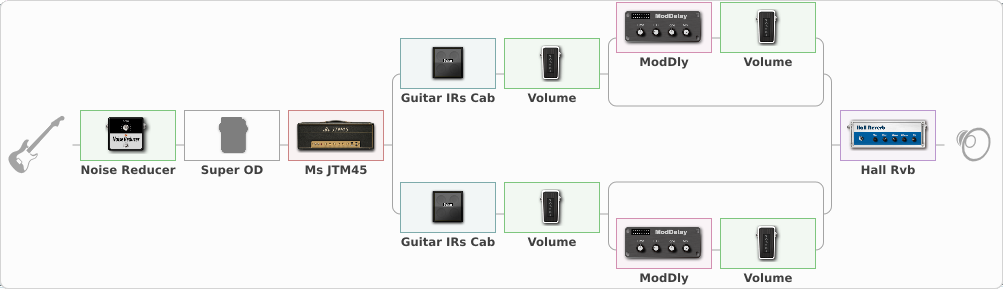# APS MJt Crunch/Solo

Discussion in 'ToneLib-GFX presets' started by Audio Pirate Studio, Apr 26, 2020.

1. ### Audio Pirate StudioNew Member

APS MJt Crunch/Solo

Preset name: APS MJt Crunch/Solo

Another preset with JTM45.
This is the complement for the JTM Clean, a mild-high overdrive sound AC/DC inspired. It include a Super OD as a booster for solo or more gain with single coil.
Best use with humbucker.
Delay added with real parallel routing with setting for a little doubled, stronger sound.
Try jamming along the Back in black backing track and you'll be surprised.

Effects chain:Effect: "Noise Reducer" (Dynamics / Filter), active - "yes"
{
"Sens" = 56
"Mode" = Soft
}

Effect: "Super OD" (Overdrive / Distortion), active - "no"
{
"Drive" = 10
"Tone" = 21
"Level" = 72
}

Effect: "Ms JTM45" (Amp simulators), active - "yes"
{
"Gain" = 64
"Bass" = 46
"Middle" = 57
"Treble" = 58
"Presence" = 75
"Master" = 82
"Level (dB)" = 0
}

Effect: "Splitter" (Dynamics / Filter), active - "yes"
{
"A-Bypass" = Off
"A-Pan" = 0
"A-Level" = 55
"B-Bypass" = Off
"B-Pan" = 0
"B-Level" = 55

'A' branch:
{

Effect: "Guitar IRs Cab" (Cabinets), active - "yes"
{
"Model" = Marshall 1960A (4x12")
"Mic Position" = Center
"Mic Distance" = Near
"Low Cut (Hz)" = 80
"Hi Cut (kHz)" = 20.0
"Mix" = 100
"Level (dB)" = 7
}

Effect: "Volume" (Dynamics / Filter), active - "yes"
{
"Pedal Pos." = 100
"Pan" = -18
}

Effect: "Splitter" (Dynamics / Filter), active - "yes"
{
"A-Bypass" = Off
"A-Pan" = 0
"A-Level" = 55
"B-Bypass" = Off
"B-Pan" = 0
"B-Level" = 55

'A' branch:
{

Effect: "ModDly" (Delay), active - "yes"
{
"Time" = Note sync, 1/8
"Feedback" = 3
"Tone" = 26
"Speed" = 0.1
"Mix" = 100
}

Effect: "Volume" (Dynamics / Filter), active - "yes"
{
"Pedal Pos." = 9
"Pan" = 20
}
}
'B' branch:
{
}
}
}
'B' branch:
{

Effect: "Guitar IRs Cab" (Cabinets), active - "yes"
{
"Model" = Marshall 1960b (4x12")
"Mic Position" = Middle
"Mic Distance" = Middle
"Low Cut (Hz)" = 89
"Hi Cut (kHz)" = 20.0
"Mix" = 100
"Level (dB)" = 7
}

Effect: "Volume" (Dynamics / Filter), active - "yes"
{
"Pedal Pos." = 100
"Pan" = 25
}

Effect: "Splitter" (Dynamics / Filter), active - "yes"
{
"A-Bypass" = Off
"A-Pan" = 0
"A-Level" = 55
"B-Bypass" = Off
"B-Pan" = 0
"B-Level" = 55

'A' branch:
{
}
'B' branch:
{

Effect: "ModDly" (Delay), active - "yes"
{
"Time" = Note sync, 1/8
"Feedback" = 5
"Tone" = 78
"Speed" = 0.1
"Mix" = 100
}

Effect: "Volume" (Dynamics / Filter), active - "yes"
{
"Pedal Pos." = 11
"Pan" = -14
}
}
}
}
}

Effect: "Hall Rvb" (Reverb), active - "yes"
{
"Time" = 4.3
"PreDelay" = 4
"LoDamp" = 2
"HiDamp" = 43
"Mix" = 27
}

Note: You will need to download and install the ToneLib-GFX software to use the preset.

File size:
1.3 KB
Views:
2,943